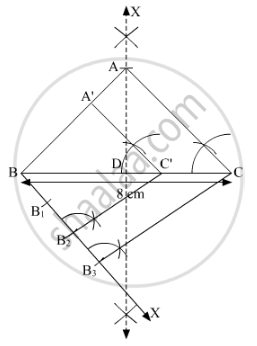Share

# Construct an Isosceles Triangle with Base 8 Cm and Altitude 4 Cm. Construct Another Triangle Whose Sides Are 2/3 Times the Corresponding Sides of the Isosceles Triangle. - CBSE Class 10 - Mathematics

#### Question

Construct an isosceles triangle with base 8 cm and altitude 4 cm. Construct another triangle whose sides are 2/3 times the corresponding sides of the isosceles triangle.

#### Solution

Steps of Construction

Step 1. Draw a line segment BC = 8 cm.

Step 2. Draw the perpendicular bisector XY of BC, cutting BC at D.

Step 3. With D as centre and radius 4 cm, draw an arc cutting XY at A.

Step 4. Join AB and AC. Here, ∆ABC is an isosceles whose base is 8 cm and altitude is 4 cm.

Step 5. Below BC, draw an acute angle ∠CBX.

Step 6. Along BX, mark three points B1, B2 and B3 such that BB1 = B1B2 = B2B3.

Step 7. Join CB3.

Step 8. From B2, draw B2C' || CB3 meeting BC at C'.

Step 9. From C', draw A'C' || AC meeting AB in A'.Here, ∆A'BC' is the required triangle similar to ∆ABC such that each side of ∆A'BC' is 2/3 times the corresponding side of ∆ABC.

Is there an error in this question or solution?

#### APPEARS IN

Solution Construct an Isosceles Triangle with Base 8 Cm and Altitude 4 Cm. Construct Another Triangle Whose Sides Are 2/3 Times the Corresponding Sides of the Isosceles Triangle. Concept: Division of a Line Segment.
S